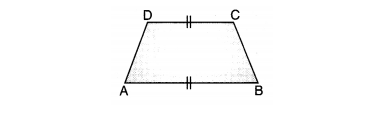Most Affordable JEE | NEET | 8,9,10 Preparation by Kota's Top IITian Doctor Faculties

# Understanding Quadrilaterals Class 8 Maths Formulas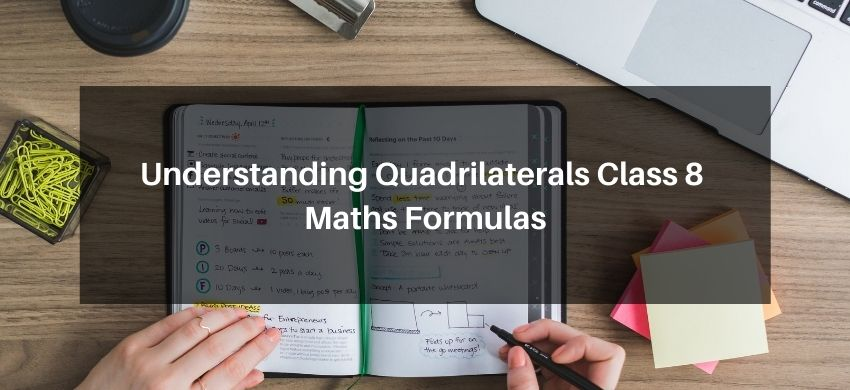`
Hey, students are you looking for Quadrilaterals Class 8 Maths Formulas? If yes. Then you are at the right place. In this post, I have listed all the formulas of Quadrilaterals class 8 that you can use to learn and understand the concepts easily.

If you want to improve your class 8 Math, Quadrilaterals concepts, then it is super important for you to learn and understand all the formulas.

With the help of these formulas, you can revise the entire chapter easily.

## Understanding Quadrilaterals Class 8 Maths Formulas

Polygon: A polygon is a closed figure bounded by three or more line segments such that each line segment intersects exactly two other points (vertices) as shown in the following figures.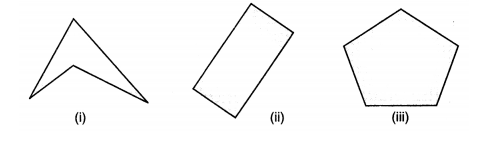Quadrilateral: A simple closed figure bounded by four line segments is called a quadrilateral, it has four sides, i.e., AB, BC, CD and AD and four vertices as A, B, C and D and the sum of all angles of a quadrilateral is 360°.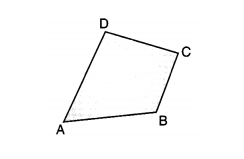Parallelogram: A quadrilateral in which opposite sides are parallel and equal is called parallelogram; written as || gm. and the diagonals of a parallelogram bisect each other.

Properties of parallelogram:
• Opposite angles are equal.
• Diagonals bisect each other.
• Opposite sides are equal and parallel.Curve: A figure formed on a plane surface by joining a number of points without lifting a pencil is called a curve.

Open Curve: A curve which does not end at the same starting point or which does not cut itself is called an open curve.

Closed Curve: A curve which cut itself or which starts and ends at the same point is called a closed curve.

Simple Closed Curve: A closed curve called a simple closed curve which does not intersect itself.

Rectangle: A parallelogram each of whose angle is 90° and diagonals are equal, is called a rectangle.

Properties:
• Opposite sides are equal and parallel.
• Each angle is a right angle.
• Diagonals are equal.
• Diagonals bisect each other.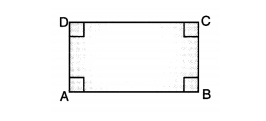Square: A quadrilateral in which all sides and angles are equal, is called a square.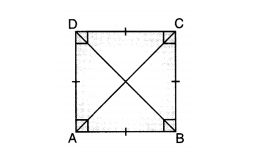Rhombus: A parallelogram having all its sides equal, is called a rhombus.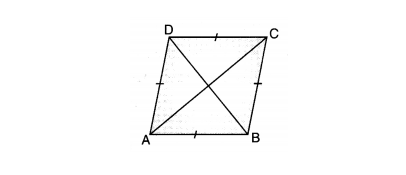Trapezium: A quadrilateral in which two opposite sides are parallel and the other two opposite sides are non-parallel, is called a trapezium. If two non-parallel sides of a trapezium are equal, then it is called an isosceles trapezium.